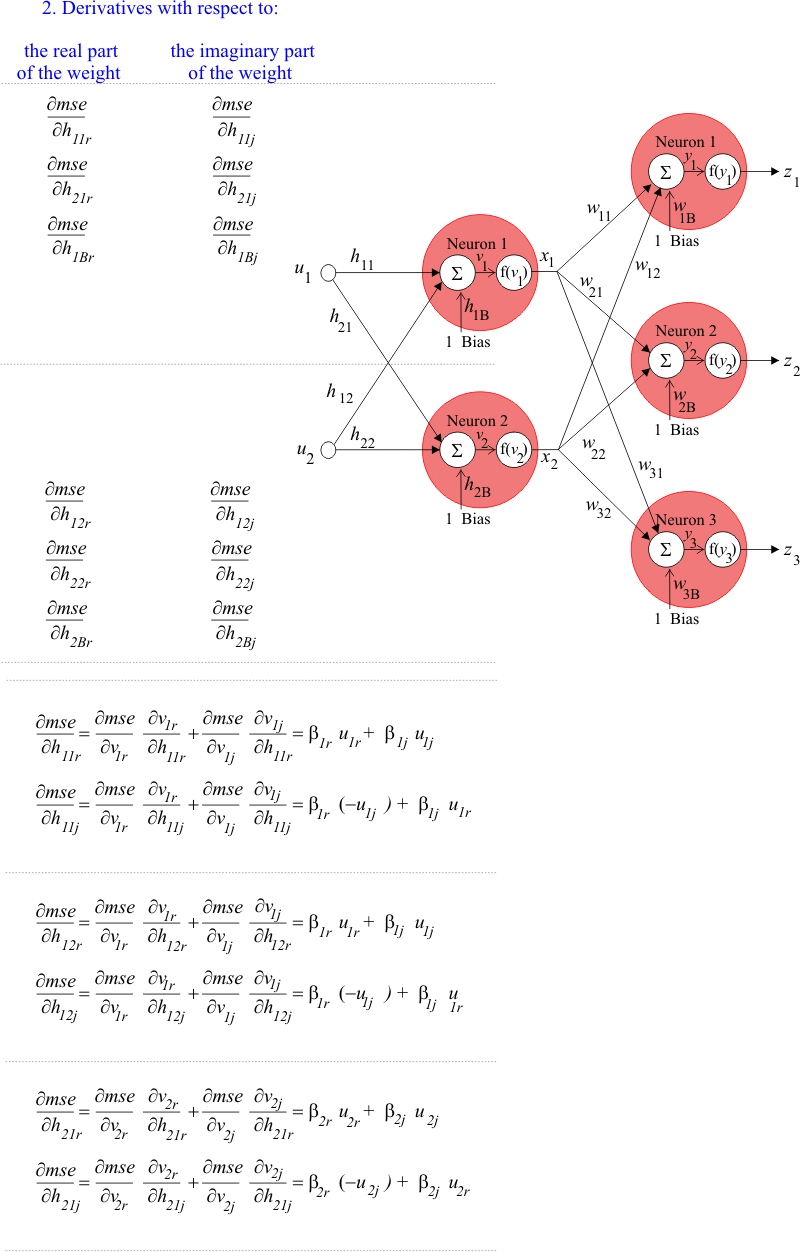# Weights Derivatives for neurons in the hidden layer

## Hidden Layer Weights

In order to compute the derivative of the mse for each weight in the hidden layer, we need to perform two steps:
1. For each neuron in the hidden layer compute the real part of β and the imaginary part of β
2. For each weight in the hidden layer compute the respective partial derivatives: one with respect the real part of the weight, and the other one with respect to the imaginary part of the weight